# SAT Math Multiple Choice Question 785: Answer and Explanation

### Test Information

Question: 785

5. Line L passes through the coordinate points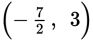and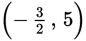. What is the slope of line L?

• A. –1
• B. -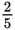• C.• D. 1

Explanation:

D

Difficulty: Easy

Category: Heart of Algebra / Linear Equations

Strategic Advice: When you're given two points on a line, find the slope using the slope formula,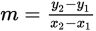. Remember, slope is rise over run, so the y's (which rhymes with "rise") go in the numerator and the x's go in the denominator.

Getting to the Answer: Be careful when you substitute the values into the formula and when you subtract negative numbers: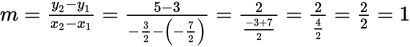The slope of line L is 1, which is (D).